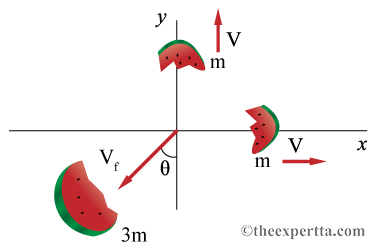# Problem: A watermelon is blown into three pieces by a large firecracker. Two pieces of equal mass m fly away perpendicular to one another, one in the x direction another in the y direction. Both of these pieces fly away with a speed of V = 25 m/s. The third piece has three times the mass of the other two pieces.Part (a) Write an expression for the speed of the larger piece, that is in terms of only the variable V.Part (b) What is the numeric value for the speed of the larger piece, in meters per second?Part (c) At what angle does the largest piece travel with respect to the -y axis, in degrees?

###### FREE Expert Solution

2D vector Magnitude & Direction:

$\overline{)\mathbf{|}\stackrel{\mathbf{⇀}}{p}\mathbf{|}{\mathbf{=}}\sqrt{{{\mathbit{p}}_{\mathbf{x}}}^{\mathbf{2}}\mathbf{+}{{\mathbit{p}}_{\mathbf{y}}}^{\mathbf{2}}}}$

$\overline{){\mathbf{tan}}{\mathbit{\theta }}{\mathbf{=}}\frac{{\mathbit{p}}_{\mathbit{y}}}{{\mathbit{p}}_{\mathbit{x}}}}$

Momentum:

$\overline{){\mathbf{p}}{\mathbf{=}}{\mathbf{m}}{\mathbf{v}}}$

(a)

Initial momentum is zero - the watermelon was at rest.

Conservation of momentum along the y-axis:

0 = (mV - (3m)(Vf)cosθ)j

Conservation of momentum along the x-axis:

0 = (mV - (3m)(Vf)sinθ)i

93% (74 ratings)###### Problem Details

A watermelon is blown into three pieces by a large firecracker. Two pieces of equal mass m fly away perpendicular to one another, one in the x direction another in the y direction. Both of these pieces fly away with a speed of V = 25 m/s. The third piece has three times the mass of the other two pieces.Part (a) Write an expression for the speed of the larger piece, that is in terms of only the variable V.

Part (b) What is the numeric value for the speed of the larger piece, in meters per second?

Part (c) At what angle does the largest piece travel with respect to the -y axis, in degrees?